CLAT  >  Alphabet - 2

# Alphabet - 2 - Logical Reasoning for CLAT

 Table of contentsAlphabet (Part 2) - Reasoning for CLAT 2018 PreparationRule 7: Relative Distance Between Pairs Of LettersRule 8: New Word FormationRule 9: Interchange Position Of Alphabet## Rule 7: Relative Distance Between Pairs Of Letters

In this type of questions, you are required to find out such pairs of letters in the given word which have as many letters between them in the word as in the alphabet.

If the word is a small one, we can just do the exercise mentally and find out the respective pairs.

In the word ‘arc’, the letters ‘a’ and ‘c’ are separated by one letter ‘r’ only; and in the English alphabet both these letters ‘a’ and ‘c’ are also separated by one letter ‘b’. From it, we conclude that there is ‘one’ such pair in the word arc, which has as much distance between them as in the English alphabet.

But, if the word has many letters in it, in such a case, we should note down their place values, because counting is easier than moving between the letters of the English alphabet.

In the word ‘SUPERSONIC’, there are ten letters, and their place values are as under: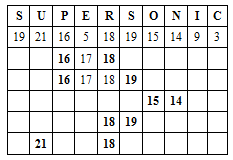So, there are five such pairs of letters.

### Now consider the more examples:

Ex 42:How many such pairs of letters are there in the word BRIGHTER which have as many letters between them in the word as in the English alphabet?

Ans 42: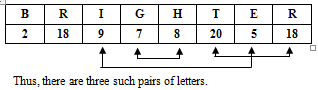Thus, there are three such pairs of letters.

Ex 43: How many such pairs of letters are there in the word DABBLE each of which has as many letters between them in the word as in the English alphabet?

Ans 43: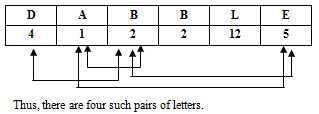Thus, there are four such pairs of letters.

Ex 44:  How many such pairs of letters are there in the word PENCIL each of which has as many letters between them as in the alphabet?

Ans 44: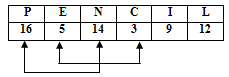Thus, there are two such pairs of letters.

Ex 45:  How many such pairs of letters are there in the word ADEQUATELY each of which has as many letters between them in the word as in the English alphabet?

Ans 45: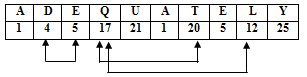Thus, there are three such pair of letters.

Ex 46: How many such pairs of letters are there in the word SURPRISE each of which has as many letters between them in the word as in the English alphabet?

Ans: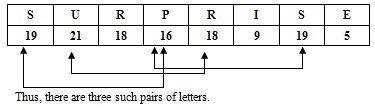Thus, there are three such pairs of letters.

## Rule 8: New Word Formation

In this type of questions, you are required to form meaningful word(s) using the specified letters of a keyword using each letter only once. Here a key word is given and some letters of that word are singled out. With the help of those letters you are required to form a new meaningful word and then, point out the required letter of the word so formed. These questions thus test your vocabulary. Generally, simple and common words are asked and, therefore, you can solve these questions easily. However, sufficient practice is necessary to save time.

Now consider the following examples:

Ex 47:  If it is possible to make only one meaningful word with the Second, the five, and the eight letters of the word “CARETAKER”, which of the following will be the first letter of that word? If more than one such word can be made, give ‘M’ as the answer and if no such word can be made, give ‘X’ as the answer.

(1) I                                            (2) N                                       (3) X                                       (4) M

Ans (4)

Solution:

 1 2 3 4 5 6 7 8 9 C A R E T A K E R

The 2nd, 5th, and the 8th letters of this word are A, T and E and following words can be formed from these letters:

 Meaningful word ⇒ EAT Meaningful word ⇒ TEA Meaningful word ⇒ ATE More than one word are formed so the answer as per the choice given is ‘M”

The 2nd, 5th, and the 8th letters of this word are A, T and E and following words can be formed from these letters:

Ex 48:  If it is possible to make only one meaningful word with the second, the fifth, and the eight and tenth letters of the word “SPONTANEOUS”, which of the following will be the first letter of that word? If more than one such word can be made, give ‘M’ as the answer and if no such word can be made, give ‘X’ as the answer.

(1) S                                        (2) O                                       (3) M                    (4) X
Ans (4)

Solution:

 1 2 3 4 5 6 7 8 9 10 11 S P O N T A N E O U S

Here, the 2nd, 5th, 8th and the 10th letters are ‘P, T, E and U’. It is not possible to make any word from these letters, so the answer here is ‘X’.

Ex 49:   If it is possible to make a meaningful word with the fourth, the seventh, the eleventh and the thirteen letters of the word CATEGORISATION, which of the following will be the second letter of that word? If more than one such word can be formed, give ‘M’ as the answer and if no such word can be formed give ‘X’ as the answer.

(1) T                                       (2) R                                       (3) X                                       (4) M

Ans (4)

 1 2 3 4 5 6 7 8 9 10 11 12 13 14 C A T E G O R I S A T I O N

The letters as per the question are ‘E, R T and O’

 Meaningful word ⇒ T O R E Meaningful word ⇒ R O T E Because, more than one word is formed from these four letters, so the answer as per option is ‘M’.

Ex 50:   If it is possible to make a meaningful word from the first, the forth, the five and the ninth letters of the word “INTERPRETATION”, last letter of the word is your answer. If more than one such word can be formed, give ‘M’ as your answer and if no such word can be formed give ‘N’ as your answer.

(1) I                                        (2) N                                       (3) N                                       (4) M

Ans (4)

 1 2 3 4 5 6 7 8 9 10 11 12 13 I N T E R P R E T A T I O

Specified letters I, E, R, T

 Meaningful word ⇒ T I E R Meaningful word ⇒ R I T E Meaningful word ⇒ T I R E More than one word is formed, so the answer is ‘N’

Ex 51:   If it is possible to make a meaningful word with the second, third, fifth and twelfth letters of the word METROPOLITAN, using each letter only once, then what is the last letter of that word? It more than one such word can be formed mark ‘M’ as the answer and it no such word can be formed mark ‘X’ as the answer.

(1) O                                       (2) E                                       (3) X                                       (4) M

Ans (4)

Solution :

 1 2 3 4 5 6 7 8 9 10 11 12 M E T R O P O L I T A N

Specified letters ⇒ E, T, O, N

 Meaningful word ⇒ N O T E Meaningful word ⇒ T O N E More than one word is formed, so the answer is ‘M’.

## Rule 9: Interchange Position Of Alphabet

Ex 52:  If the new word is formed such as the new word starts with last four letters of the word CONCENTRATION  written in reverse order followed by next two in reverse order, then the next three in reverse order and then followed by first four in the reverse order, which letter would be eighth from the right end?

 C O N C E N T R A T I O N N O I T A R T N E C N O C

It is clear from diagram that R is at 8th position from the right end.

Ex 53: If the 1 & 3, 2 & 4, 5 & 7, 6 & 8 letters of the word PLEASURE are interchanged in the word , which will be second from right?

Solution :  There are eight letters in the word ‘PLEASURE’ and the  second letter from right will be 7th letter from left side. The 7th letter is getting exchanged with the 5th letter, so the 5th letter ‘S’ will now occupy the place at present occupied by R.

 P L E A S U R E E A P L R E S U

It is clear from diagram that 2nd from the right is S.

Ex 54:  If the first and second letters of the word ‘DEPRESSION’ were interchanged, also the third and fourth letters the fifth and the sixth letters and so on, which of the following would be the seventh letter from the right?

Solution: There are ‘eleven’ letters in the word and the ‘seventh’ from right will be ‘fifth’ from left. The fifth letter is getting exchanged with the ‘fourth’ letter ‘P’, so the letter P will occupy the seventh place  from right side.

 D E P R E S S I O N E D R P S E I S N O

Ex 55:  If position of 5th & 12th, 4th & 14th, 3rd & 10th, 2nd & 11th, 1st & 13th. if the word “GLORIFICATIONS”  are interchanged. What will be 12th letter from right end?

Sol.  We want the position 12th from right end. There are ‘fourteen’ letters in this word, so the 12th letter from right means 15 – 12= 3rd from left side.

But 3rd letter is interchanged with 10th letter so the answer is ‘T’.

Ex 56: In the word, “GLORIFICATIONS”, if the first letter is changed with last letter, second letter with second last and so on. Which letter will come after T in the new arrangement?

Sol:  After T, comes ‘A’. The result of these changes will be that the whole sequence of alphabets will be reversed. So, now A will come after ‘T’.

The document Alphabet - 2 | Logical Reasoning for CLAT is a part of the CLAT Course Logical Reasoning for CLAT.
All you need of CLAT at this link: CLAT

## FAQs on Alphabet - 2 - Logical Reasoning for CLAT

 1. What is the rule of relative distance between pairs of letters in the context of alphabet reasoning for CLAT 2018 preparation?Ans. The rule of relative distance between pairs of letters refers to the concept of analyzing the position of letters in relation to each other. It involves understanding the distance or number of letters between two given letters in the alphabet. This rule is important in solving alphabet reasoning questions and can help determine the pattern or sequence followed in a given set of letters.
 2. How does new word formation relate to alphabet reasoning in CLAT 2018 preparation?Ans. New word formation is a rule used in alphabet reasoning to create new words or sequences by rearranging or modifying the given letters. It involves rearranging the letters in a specific pattern or order to form a meaningful word or series. Understanding this rule helps in solving questions that require finding the missing letter or word based on a given pattern or sequence.
 3. What does it mean to interchange the position of the alphabet in alphabet reasoning for CLAT 2018 preparation?Ans. Interchanging the position of the alphabet refers to swapping or exchanging the places of two or more letters in a given sequence. This rule is often used in alphabet reasoning questions to create a new pattern or sequence by rearranging the letters. By interchanging the position of the alphabet, one can identify the logic or pattern followed and solve the given question.
 4. How can the rule of relative distance between pairs of letters be applied in solving alphabet reasoning questions for CLAT 2018 preparation?Ans. To apply the rule of relative distance between pairs of letters, one must analyze the given set of letters and identify the position of each letter in relation to the others. By observing the distances or number of letters between specific pairs, one can determine the pattern or sequence followed. This rule helps in finding missing letters, predicting the next letter in a series, or identifying the logic behind the given set of letters.
 5. Can you provide an example of a question that involves the rule of new word formation in alphabet reasoning for CLAT 2018 preparation?Ans. Sure! Here's an example: Question: In the given series of letters - ABDE, BCDF, CEGH, DEIJ, what would be the next letter sequence? Answer: The given series follows the rule of new word formation, where each letter is incremented by one position in the alphabet. Applying the same rule, the next letter sequence would be EFIK.

## Logical Reasoning for CLAT

19 videos|27 docs|67 tests

## Logical Reasoning for CLAT

19 videos|27 docs|67 tests
Signup to see your scores go up within 7 days! Learn & Practice with 1000+ FREE Notes, Videos & Tests.
10M+ students study on EduRev
Track your progress, build streaks, highlight & save important lessons and more!(Scan QR code)
Related Searches

,

,

,

,

,

,

,

,

,

,

,

,

,

,

,

,

,

,

,

,

,

;